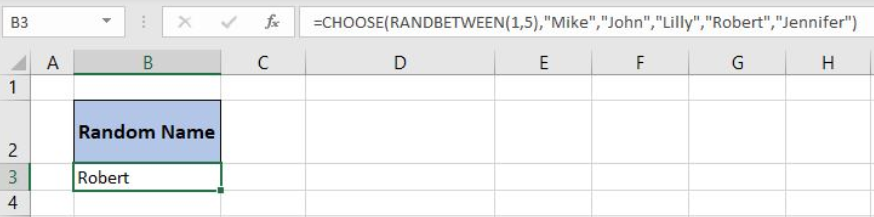Go Back

# Here is How You Can Randomly Generate Words in Excel

Excel allows us to get a random word from a range using the CHOOSE and  RANDBETWEEN functions. This step by step tutorial will assist all levels of Excel users to learn how to randomly generate words in Excel.xFigure 1. The final result of the formula

## Syntax of the RANDBETWEEN Formula

The generic formula for the RANDBETWEEN function is:

`=RANDBETWEEN(bottom, top)`

The parameters of the RANDBETWEEN function are:

• bottom – a value from which we want to get a random value
• top – a value to which we want to get a random value.

## Syntax of the CHOOSE Formula

The generic formula for the CHOOSE function is:

`=CHOOSE(index_num, value1, [value2], [value3],...)`

The parameters of the CHOOSE function are:

• index_num – an index of the value which we want to retrieve
• value1, [value2], [value3],… – a list of values from which one value is returned.

## Get a Random Word Using the Formula

In the cell B3, we want to get a random name from the name list: “Mike”, “John”, “Lilly”, “Robert” and “Jennifer”.

The formula looks like:

`=CHOOSE(RANDBETWEEN(1, 5), "Mike","John","Lilly","Robert","Jennifer")`

The parameter bottom of the RANDBETWEEN function is 1 and the top is 5. The result of this function is a random number between 1 and 5 which is the index_num parameter of the CHOOSE function. The values of the function are “Mike”, “John”, “Lilly”,” Robert”, “Jennifer”.

To apply the formula, we need to follow these steps:

• Select cell B3 and click on it
• Insert the formula: `=CHOOSE(RANDBETWEEN(1, 5), "Mike","John","Lilly","Robert","Jennifer")`
• Press enter.

19483Figure 2. Using the formula to get a random word from the range

If we evaluate the function, we can see that the RANDBETWEEN function returns number 4. Therefore, the CHOOSE function returns the 4th value from the values. Finally, the result in the B3 cell is “Robert”.

Most of the time, the problem you will need to solve will be more complex than a simple application of a formula or function. If you want to save hours of research and frustration, try our live Excelchat service! Our Excel Experts are available 24/7 to answer any Excel question you may have. We guarantee a connection within 30 seconds and a customized solution within 20 minutes.

### Did this post not answer your question? Get a solution from connecting with the expert.

Another blog reader asked this question today on Excelchat: